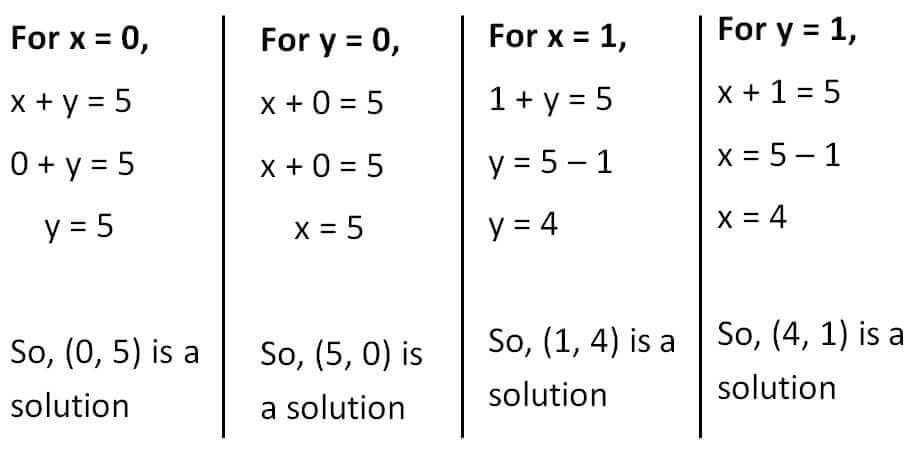Solution of linear equation

Chapter 4 Class 9 Linear Equations in Two Variables
Concept wise

Values of x and y which satisfy the linear equation are called solutions of linear equation.

Let us take linear equation

x + y = 5

We find 4 different solutions of the linear equationx 0 5 1 4 y 5 0 4 1

So four solutions of the given equation are:

(0, 5), (5, 0) ,  (1, 4) and (4, 1).

Similarly, we can get many solutions

For a linear equation, there are infinitely many solutions.

Learn in your speed, with individual attention - Teachoo Maths 1-on-1 Class